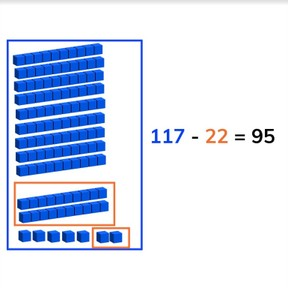Subtraction through 100 regrouping hundreds as tens

# Subtraction through 100 regrouping hundreds as tens

Subtract numbers to 100 regrouping hundreds as tens.

No account needed.8,000 schools use Gynzy92,000 teachers use Gynzy1,600,000 students use Gynzy

## General

Students learn to subtract numbers to 100 regrouping hundreds as tens.

2.NBT.B.5

## Relevance

It is important to be able to subtract numbers through 100, so you can determine how many you have left.

## Introduction

Start by practicing with placing numbers on the number line. Ask a student to come to the front and to drag the given numbers to where they belong on the number line. Then practice solving a subtraction problem on the number line.

## Development

Start by discussing the importance of subtracting through one hundred. Next the learning goal is given in three ways, visually, in the abstract, and as story problems. You can navigate beween these three by using the blue menu on the bottom right. You can select the method which best meets the learning needs of your class. The lesson starts with the visual method. Using the blocks on the interactive whiteboard explain how to solve a subtraction problem. Have students practice subtraction problems with visual support. Then discuss the abstract subtraction problems. Tell students that they can solve these problems by counting back or by counting back in jumps. Using a number line can be very helpful here. Have students practice abstract problems individually or in pairs. Finally discuss the steps of solving a story problem, namely that they must determine what kind of math problem it is, which numbers are important for the problem, to say or write the problem and then to solve the problem. Solve one story problem as a class and then have students solve a story problem individually or in pairs.

Check that students are able to subtract numbers through 100 regrouping hundreds as tens by asking the following questions:
- Why is it useful to be able to subtract through 100?
- What do you do with the second number in a subtraction problem (the subtrahend)?

## Guided practice

Students first practice with problems with visual support and then with abstract and finally in story forms. All types of questions are present in the guided practice.

## Closing

Ask students to close the lesson by solving a visual, abstract and story problem individually or in pairs. Have students explain their strategy.

## Teaching tips

Students who have difficulty with this learning goal can be supported by the use of manipulatives or by using the number line to draw the jumps they take away from the minuend (first number).

## Instruction materials

Optional: MAB blocks or other maniplulatives

### The online teaching platform for interactive whiteboards and displays in schools

• Save time building lessons

• Manage the classroom more efficiently

• Increase student engagement